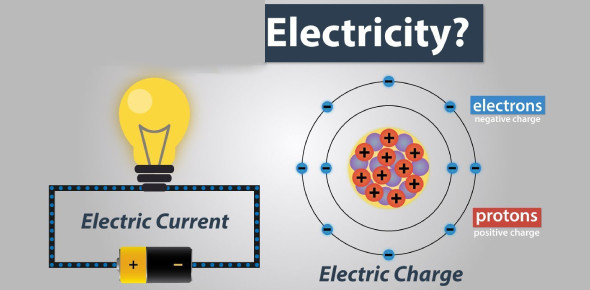# 9th Grade Quiz On Electricity! Trivia Test

13 Questions | Total Attempts: 202SettingsWould you like to try this 9th Grade quiz on electricity? This quiz will show you why it is essential to learn about electricity. You must recognize the definition of a renewable resource and give an example of current electricity measured by volts and protons. This quiz is perfect for those kids who are interested in learning about electricity. Do you think you can pass this quiz? Take the quiz and prove your knowledge.

• 1.
What is the definition of a renewable resource AND pick an example?
• A.

Resources that can be continually reproduced over a relatively short period of time

• B.

Natural resources that cannot be replaced once they are used up

• C.

Hydrogen Energy

• D.

Uranium

• 2.
What is current electricity?
• A.

Continuous flow of electrons in a circuit

• B.

A charge that is built up then dispersed

• C.

Energy stored within an item, that it then released

• 3.
Unlike  ___________________, current electricity needs an energy source and a complete path to follow.
• 4.
_________________ is the degree to which a substance resists the flow of electric current through it. All substances resist electron flow to some extent.
• 5.
An _______ is a device for measuring resistance.
• 6.
What is measured by volts.
• A.

Current

• B.

Potential Difference

• C.

Resistance

• D.

Electricity

• E.

None of the above

• 7.
What is a proton
• A.

A positively charged particle

• B.

A negatively charged particle

• C.

A neutral particle

• D.

An atom

• E.

None of these

• 8.
_____________ can flow when there is a complete circuit.
• 9.
Materials through which electricity can pass are called ___________ conductors.
• 10.
____________ is the movement of electrons within a substance caused by nearby charged objects, without direct contact between the substance and the object.
• 11.
What happens when a switch is turned off?
• A.

Electricity stops flowing from the switch and therefore the circuit is broken, providing no electricity to the object being powered

• B.

Electricity is able to flow through the circuit powering an electrical device

• C.

Electricity is not able to flow through the circuit but it can still power an electrical device

• D.

Electricity is able to flow through the circuit but it cannot power an electrical device

• 12.
Which one is the odd one out?
• A.

Glass

• B.

Tin

• C.

Copper

• D.

Silver

• 13.
What is in an atom?
• A.

Protons, Electrons, Neutron and a Nucleus

• B.

Protons and Electrons

• C.

A nucleus and a proton

• D.

Protons and Neutrons

• E.

Protons, Electrons Neutron

Related TopicsBack to top